# Tomita-Takesaki theory

(Redirected from Tomita–Takesaki theory)
Jump to: navigation, search

M. Tomita [a4] defined the notion of a left Hilbert algebra as follows: An involutive algebraover the fieldof complex numbers, with involution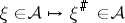, that admits an inner product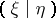satisfying the following conditions:

i) the mapping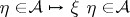is continuous for every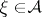;

ii)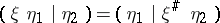for all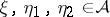;

iii)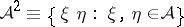is total in the Hilbert space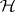obtained by completion of.

iv)is a closeable conjugate-linear operator in. Letbe a left Hilbert algebra in a Hilbert space. For any, let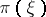denote the unique continuous linear operator onsuch that,. The von Neumann algebra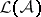generated by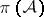is called the left von Neumann algebra of. Letbe the closure of the mapping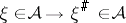and letbe the polar decomposition of. Thenis an isometric involution andis a non-singular positive self-adjoint operator insatisfying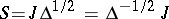and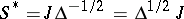;and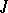are called the modular operator and the modular conjugation operator of, respectively. Let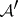denote the set of vectors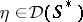such that the mapping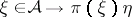is continuous. For any, denote by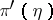the unique continuous extension of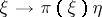to. Let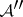be the set of vectorssuch that the mapping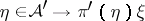is continuous. For any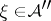, denote bythe unique continuous extension ofto. Thenis a left Hilbert algebra in, equipped with the multiplication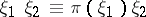and the involution, andis equivalently contained in, that is,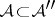and they have the same modular (conjugation) operators. The set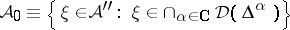is a left Hilbert algebra which is equivalently contained inandis a complex one-parameter group of automorphisms of, called the modular automorphism group. It satisfies the conditions:

a)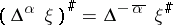,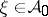,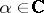;

b),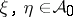,;

c)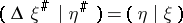,;

d)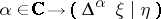,, is an analytic function on. Such a left Hilbert algebra is called a modular Hilbert algebra (or Tomita algebra). Using the theory of modular Hilbert algebras, M. Tomita proved that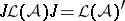and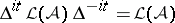for all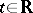. This theorem is called the Tomita fundamental theorem. M. Takesaki [a3] arranged and deepened this theory and connected this theory with the Haag–Hugenholtz–Winnink theory [a2] for equilibrium states for quantum statistical mechanics. After that, Tomita–Takesaki theory was developed by A. Connes [a1], H. Araki, U. Haagerup and the others, and has contributed to the advancement of the structure theory of von Neumann algebras, non-commutative integration theory, and quantum physics. Using an integral formula relating the resolvent of the modular operatorwith the operators, A. van Daele [a5] has simplified a discussion in the complicated Tomita–Takesaki theory.